# GSEB Solutions Class 8 Maths Chapter 9 Algebraic Expressions and Identities Ex 9.1

Gujarat Board GSEB Textbook Solutions Class 8 Maths Chapter 9 Algebraic Expressions and Identities Ex 9.1 Textbook Questions and Answers.

## Gujarat Board Textbook Solutions Class 8 Maths Chapter 9 Algebraic Expressions and Identities Ex 9.1

Question 1.
Identify the terms, their coefficients for each of the following expressions?

1. 5xyz2 – 3zy
2. 1 + x + x2
3. 4x2y2 – 4x2y2z2 + z2
4. 3 – pq + qr – rp
5. $$\frac{x}{2}$$ $$\frac{y}{2}$$ xy
6. 0.3a – 0.6 ab + 0.5b

Solution:
1. 5xyz2 – 3zy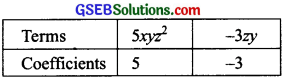2. 1 + x + x2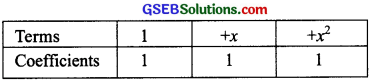3. 4x2y2 – 4x2y2z2 + z2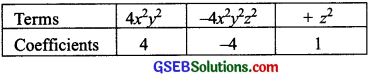4. 3 – pq + qr – rp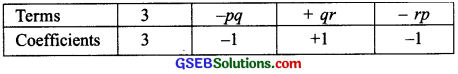5. $$\frac{x}{2}$$ + $$\frac{y}{2}$$ – xy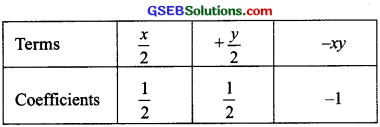6. 0.3a – 0.6ab + 0.5bQuestion 2.
Classify the following polynomials as monomials, binomials, trinomials. Which polynomials do not fit in any of these three categories?
x + y, 1000, x + x2 + x3 + x4, 7 + y + 5x, 2y – 3y2, 2y – 3y2, 2y – 3y2 + 4y3, 5x – 4y + 3xy, 4z – 15z2, ab + bc + cd + da, pqr, p2q + pq2, 2p + 2q
Solution:Following polynomials do not fit in any of these categories:
x + x2 + x3 + x4 [∵ It has 4 terms]
ab + bc + cd + da [∵It has 4 terms]

Question 3.
(i) ab – bc, bc – ca, ca – ab
(ii) a – b + ab, b – c + bc, c – a + ca
(iii) 2p2q2 – 3pq + 4, 5 + 7pq – 3p2q2
(iv) l2 + m2, m2 + n2, n2, n2 + l2, 2lm + 2mn + 2nl
Solution:Question 4.
(a) Subtract 4a – 7ab + 3b + 12 from 12a – 9ab + 5b – 3
(b) Subtract 3xy + 5yz – 7zx from 5xy – 2yz – 2zx + 10xyz
(c) Subtract 4p2q – 3pq + 5pq2 – 8p + 7q – 10 from
18 – 3p – 11q + 5pq – 2pq2 + 5p2q.
Solution:
For subtraction we rearrange the terms such that the like terms are in the same column. Then we change the sign of the terms to be substracted. Next we add the terms.